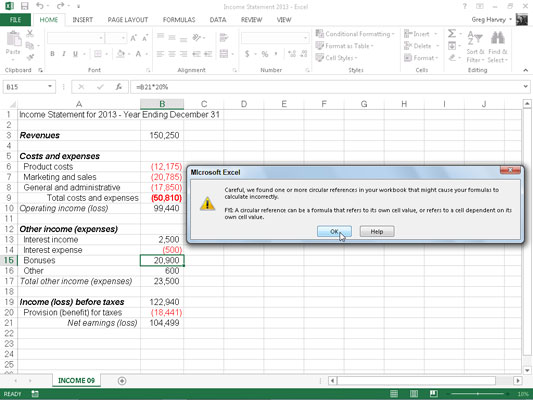##### Excel 2013 All-in-One For DummiesA circular reference in an Excel 2013 formula is one that depends, directly or indirectly, on its own value. The most common type of circular reference occurs when you mistakenly refer in the formula to the cell in which you’re building the formula itself. For example, suppose that cell B10 is active when you build this formula:

`=A10+B10`

As soon as you click the Enter button on the Formula bar or press Enter or an arrow key to insert this formula in cell B10 (assuming the program is in Automatic recalculation mode), Excel displays an Alert dialog box, stating that it cannot calculate the formula due to the circular reference.

If you then press Enter or click OK to close this Alert dialog box, an Excel Help window appears containing general information about circular references in two sections: Locate and Remove a Circular Reference and Make a Circular Reference Work by Changing the Number of Times Microsoft Excel Iterates Formulas.

When you close this Excel Help window by clicking its Close button, Excel inserts 0 in the cell with the circular reference, and the Circular Reference status indicator followed by the cell address with the circular reference appears on the status bar.

Some circular references are solvable by increasing the number of times they are recalculated (each recalculation bringing you closer and closer to the desired result), whereas others are not (for no amount of recalculating brings them closer to any resolution) and need to be removed from the spreadsheet.

The formula in cell B10 is an example of a circular reference that Excel is unable to resolve because the formula’s calculation depends directly on the formula’s result. Each time the formula returns a new result, this result is fed into the formula, thus creating a new result to be fed back into the formula.

Because this type of circular reference sets up an endless loop that continuously requires recalculating and can never be resolved, you need to fix the formula reference or remove the formula from the spreadsheet.

The figure illustrates the classic example of a circular reference, which ultimately can be resolved. Here, you have an income statement that includes bonuses equal to 20 percent of the net earnings entered as an expense in cell B15 with the formula`=–B21*20%`

This formula contains a circular reference because it refers to the value in B21, which itself indirectly depends on the amount of bonuses (the bonuses being accounted for as an expense in the very worksheet formulas that determine the amount of net earnings in cell B21).

To resolve the circular reference in cell B15 and calculate the bonuses based on net earnings in B21, you simply need to select the Enable Iterative Calculation check box in the Calculation Options section of the Formulas tab in the Excel Options dialog box (File→Options→Formulas or Alt+FTF).

However, if manual recalculation is selected, you must click the Calculate Now (F9) command button on the Formulas tab of the Ribbon or press F9 or Ctrl+= as well.